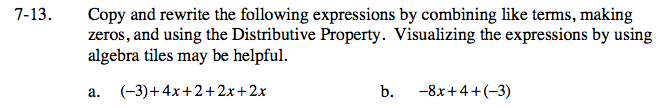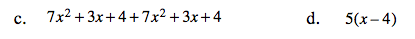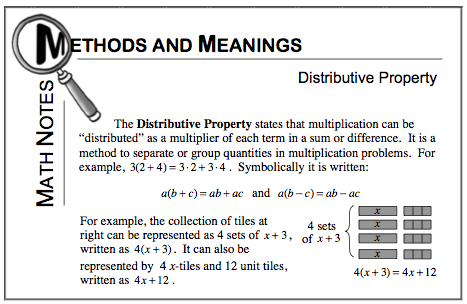Home > CC2MN > Chapter cc27 > Lesson cc27.1.1 > Problem7-13

7-13.
1. Copy and rewrite the following expressions by combining like terms, making zeros, and using the Distributive Property. Visualizing the expressions by using algebra tiles may be helpful. Homework Help ✎

1. (–3) + 4x + 2 + 2x + 2x

2. –8x + 4 + (–3)

3. 7x2 + 3x + 4 + 7x2 + 3x + 4

4. 5(x – 4)Group like terms together.
(4x + 2x + 2x) + (−3 + 2)

Simplify.

8x − 1

Visualize eight negative x algebra tiles. What happens when you add 4 unit tiles but then take 3 of these unit tiles away?

−8x + 1See part (a).

Refer to the Math Notes below.